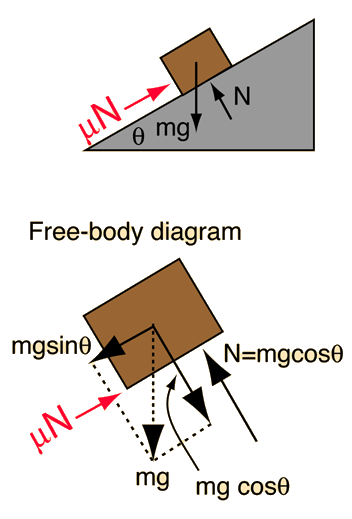# Mass on Incline With FrictionApplication of Newton's second law to mass on incline.
For an incline at angle degrees and coefficient of friction mu=, the acceleration of the object down the incline is

### Acceleration =m/s2

compared to 9.8 m/s² for freefall. Gravity would give it an acceleration of m/s² down the incline in the absence of friction, but frictional drag subtracts m/s2. If the height of the incline is h=m, then the time to slide down the incline from rest would be t=seconds, compared to a time of t=seconds for a frictionless incline and t=to drop from that height . The speed at the bottom of the incline would be m/s compared to v=m/s for a frictionless incline.

### Expressions****Remove friction

Index

Newton's laws

Standard mechanics problems

 HyperPhysics***** Mechanics R Nave
Go Back